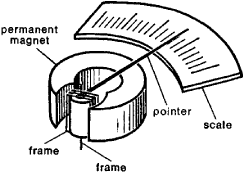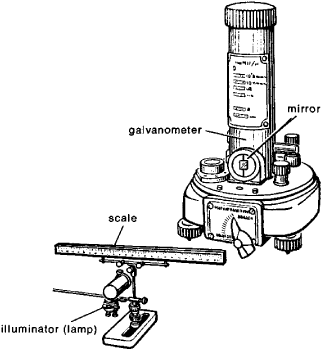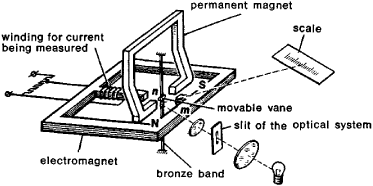# galvanometer

(redirected from D'Arsonval movement)
Also found in: Dictionary, Thesaurus, Medical.
Related to D'Arsonval movement: galvanometer, D'Arsonval meter

## galvanometer

galvanometer (gălˌvənŏmˈətər), instrument used to determine the presence, direction, and strength of an electric current in a conductor. All galvanometers are based upon the discovery by Hans C. Oersted that a magnetic needle is deflected by the presence of an electric current in a nearby conductor. When an electric current is passing through the conductor, the magnetic needle tends to turn at right angles to the conductor so that its direction is parallel to the lines of induction around the conductor and its north pole points in the direction in which these lines of induction flow. In general, the extent to which the needle turns is dependent upon the strength of the current. In the first galvanometers, a freely turning magnetic needle was hung in a coil of wire; in later versions the magnet was fixed and the coil made movable. Modern galvanometers are of this movable-coil type and are called d'Arsonval galvanometers (after Arsène d'Arsonval, a French physicist). If a pointer is attached to the moving coil so that it passes over a suitably calibrated scale, the galvanometer can be used to measure quantitatively the current passing through it. Such calibrated galvanometers are used in many electrical measuring devices. The DC ammeter, an instrument for measuring direct current, often consists of a calibrated galvanometer through which the current to be measured is made to pass. Since heavy currents would damage the galvanometer, a bypass, or shunt, is provided so that only a certain known percentage of the current passes through the galvanometer. By measuring the known percentage of the current, one arrives at the total current. The DC voltmeter, which can measure direct voltage, consists of a calibrated galvanometer connected in series (see electric circuit) with a high resistance. To measure the voltage between two points, one connects the voltmeter between them. The current through the galvanometer (and hence the pointer reading) is then proportional to the voltage (see Ohm's law).

## Galvanometer

A device for indicating very small electric currents. Although the deflection of a galvanometer results from current in the moving coil, the voltage in a closed circuit producing this current is frequently the quantity of interest to the user. In this mode, galvanometers are used to detect a null or an unbalanced condition in a bridge or potentiometer circuit. Electronic instruments that employ amplifying circuits to achieve sensitivities approaching the nanovolt level are also used as balance detectors in bridge circuits. However, in applications where extreme sensitivity and high rejection of ac signals are required, a galvanometer in combination with a photocell amplifier is to be preferred.

Galvanometers may also be used ballistically to integrate a transient current, as from the discharge of a capacitor, or a transient voltage, as produced when a coil moves relative to a magnetic field. See Current measurement, Voltage measurement

The d'Arsonval galvanometer is the most common type and is widely used. Its indicating system consists of a light coil of wire suspended from a copper or gold ribbon a few thousandths of an inch wide and less than 0.001 in. (0.025 mm) thick. This coil, free to rotate in the radial field between the shaped pole pieces of a permanent magnet, carries a small mirror which serves as an optical pointer and indicates the coil position by reflecting a light beam onto a fixed scale. Current is conducted to and from the coil by the suspension ribbons. The torque which deflects the indicating element is produced by the reaction of the coil current with the magnetic field in which it is suspended.

Voltage sensitivity is of importance in applications where the galvanometer serves as the detector of unbalance in a bridge or potentiometer network. The energy of motion in the indicating system must be dissipated and the system brought to rest in order for the deflection to be evaluated. This process, common to all indicating systems in which equilibrium must be achieved between a driving torque and a restoring torque, is called damping. See Damping

The sensitivity of modern galvanometers ranges up to 0.04 in. (1 mm) of deflection, on a scale 40 in. (1 m) distant from the mirror, for a current of 0.00001 microampere. Such a galvanometer may have a coil resistance of 800 ohms and a critical damping resistance of 100,000 &OHgr;. The voltage response of this instrument amounts to 0.04 in./microvolt (1 mm/μV) at critical damping. A galvanometer designed for voltage sensitivity, has a coil resistance of 20 &OHgr;, a critical damping resistance of 30 &OHgr;, and a response of 0.04 in. (1 mm) for 0.05 μV in the critically damped circuit. It will be seen from these examples that a large response to current is associated with large coil resistance and high critical damping resistance, whereas voltage response is associated with low coil resistance and low critical damping resistance.

The limitations reached in improving a galvanometer's resolution by increasing its scale length or using multiple reflections can be overcome by operating the galvanometer in its equilibrium position by using a negative-feedback network. In this system the beam of light reflected from the galvanometer mirror is directed onto a pair of photocells connected in series opposition (see illustration). As the galvanometer coil rotates, the light received on one photocell increases while the other decreases, and an amplified output current is produced whose sign and magnitude depend on the direction and amount of the coil deflection, respectively. The photocell output current is monitored by an external indicator and is approximately 1800 times the galvanometer input current. This photocell output also provides a negative-feedback current to servo the galvanometer at its equilibrium position, so that the linearity and input resistance of the system are increased. In such an arrangement it is frequently possible to approach rather closely the theoretical limit of resolution of angular motion imposed by the brownian motion of the coil, or by the Johnson noise of the circuit connected to the galvanometer. In a low-resistance galvanometer this theoretical resolution may be about 0.001 μV. See Brownian movement, Kinetic theory of matter

A galvanometer is an extremely efficient low-pass filter, and it is still the most sensitive low-level detector at input impedances of 1 k&OHgr; or less. At higher input impedances, chopper-stabilized amplifiers, called nanovoltmeters, exhibit better resolution, noise, and drift characteristics than galvanometers. These instruments modulate the input dc signal by using a chopper (mechanical contact, transistor, or photocell switching), then amplify the signal by traditional ac techniques to achieve sensitivities of 10 nV or less. State-of-the-art digital voltmeters are now available with nanovolt resolution and are useful as low-level detectors. See Ammeter, Electrical measurements, Electrometer, Voltmeter

McGraw-Hill Concise Encyclopedia of Physics. © 2002 by The McGraw-Hill Companies, Inc.
The following article is from The Great Soviet Encyclopedia (1979). It might be outdated or ideologically biased.

## Galvanometer

a highly sensitive electrical measuring instrument that responds to very low current or voltage values. Galvanometers are most commonly used as zero current indicators—that is, devices to indicate the absence of current or voltage in an electrical circuit. After preliminary determination of the constant for an instrument (the value of a scale division), they are also used to measure small currents and voltages.

Both DC and AC galvanometers exist. The first DC galvanometers were made in the 1820’s and operated on the principle of the permanent-magnet system of instruments. They consist of a magnetic needle suspended on a fine thread within a coil of wire. When no current flows in the coil, the needle orients itself along the local magnetic meridian. The appearance of a current causes the needle to deviate from its initial position. In the 19th century a great variety of galvanometer designs with a movable magnetic needle were developed and were extensively utilized for scientific studies of electromagnetic phenomena. For example, in 1886, F. Kohlrausch used such an instrument to determine with great accuracy the electrochemical equivalent of silver.

In 1881 the French scientist J. A. d’Arsonval created a suspended-coil galvanometer, in which the moving part is a current-carrying coil located in the field of a permanent magnet. Depending on the design of the moving part of such galvanometers, they are classified as frame types, in which the moving part is a frame with several turns of wire; loop types, in which the moving part is a single-turn wire loop; and string types, in which the moving part is a wire stretched like a string. An example of a frame galvanometer is shown in Figure 1. A frame positioned in the field of a permanent magnet has an indicating pointer attached to its axis. Current flowing through the turns of the frame interacts with the permanent magnetic field and produces a torque that causes the movable part to turn and move the pointer correspondingly along the scale. To increase sensitivity, the pointer of such a galvanometer is replaced by the miniature mirror of an optical indicating device. (This is shown in Figure 2.) A beam of light from the illuminator falls on a mirror and, upon being reflected from it, arrives at the scale. The scale is placed at a distance of 1.5 to 2 m from the galvanometer, so that even very small angular displacements of the mirror produce noticeable deflections of the luminous spot on the scale from its zero position. Galvanometers with an illuminated scale, in which the illuminator and the scale are located in the same housing with the galvanometer mechanism, are a version of this type of galvanometer. To obtain sufficient length of the light beam, it undergoes multiple reflection from several fixed mirrors.Figure 1. Frame galvanometer

When a current pulse of short duration passes through a galvanometer’s winding, the moving part is ballistically deflected from the zero position and subsequently returns to this position after several oscillations. If the pulse duration is substantially shorter than the period of the moving part’s natural oscillations, the first and greatest deflection of the indicator is proportional to the amount of electricity carried by the pulse. To measure the amount of electricity for relatively long pulses, ballistic galvanometers are made, in which the moment of inertia of the moving part is much greater than in the ordinary types. With ballistic galvanometers it is possible to measure the amount of electricity in pulses lasting up to 2 sec.

For the detection of small alternating currents and voltages, AC vibration galvanometers and galvanometers with AC-to-DC transformers are used. The operating principle of vibration galvanometers is the same as for DC galvanometers; the two types differ only in that the moment of inertia of the moving part in vibration galvanometers is very small. The mechanism of a vibration galvanometer with a moving magnet is shown in Figure 3. A movable vane made of magnetically soft steel is located between the poles of a permanent magnet and in the field of an electromagnet (between poles n and m). The vane, together with a small mirror, is attached to a bronze band. The alternating current being measuredFigure 2. Mirror galvanometer

passes through a winding on the electromagnet and creates an alternating magnetic field, which is superimposed on the constant magnetic field from the permanent magnet. The resultant field changes its direction with the frequency of the alternating current and causes the vane to vibrate; the sharp image of the illuminated slit on the scale thereby becomes blurred into a luminous band. The width of the band is proportional to the strength of the alternating current in the winding of the electromagnet. The maximum sensitivity of a vibration galvanometer is produced when the natural vibration frequency of the movable parts equals the frequency of the alternating current, and for this reason all such galvanometers have devices for changing the natural vibration frequency so that the moving part can be tuned to resonate with the test current. These types of galvanometers are manufactured for operation up to frequencies no higher than 5 kilohertz (kHz).Figure 3. Vibration galvanometer

A thermogalvanometer is an AC galvanometer with a thermoconverter. The galvanometer has the mechanism of a permanent-magnet galvanometer, with a single-turn moving frame. The halves of this turn are made of different metals, forming a thermocouple. A heater located close to one of the junctions carries the alternating current to be measured. The thermocurrent generated in the frame deflects it from the zero position. This galvanometer can be operated at frequencies above 5 kHz.

The basic characteristic of a galvanometer is its sensitivity or its inverse—the galvanometer constant. Modern mass-produced DC galvanometers can detect currents of about 5 × 10-11 amperes and voltages on the order of 5 × 10-8 volts. The constants of AC vibration galvanometers are on the order of 1 × 10-7 amperes per division.

### REFERENCES

Cherdantseva, Z. V. Elektricheskie izmereniia, 3rd ed. Moscow-Leningrad, 1933.
Karandeev, K. B. Gal’vanometry postoiannogo toka. L’vov, 1957.
Arutiunov, V. O. Elektricheskie izmeritel’nye pribory i izmereniia. Moscow, 1958.

N. G. VOSTROKNUTOV

The Great Soviet Encyclopedia, 3rd Edition (1970-1979). © 2010 The Gale Group, Inc. All rights reserved.

## galvanometer

[‚gal·və′näm·əd·ər]
(engineering)
An instrument for indicating or measuring a small electric current by means of a mechanical motion derived from electromagnetic or electrodynamic forces produced by the current.
McGraw-Hill Dictionary of Scientific & Technical Terms, 6E, Copyright © 2003 by The McGraw-Hill Companies, Inc.

## galvanometer

any sensitive instrument for detecting or measuring small electric currents
Collins Discovery Encyclopedia, 1st edition © HarperCollins Publishers 2005
Site: Follow: Share:
Open / Close